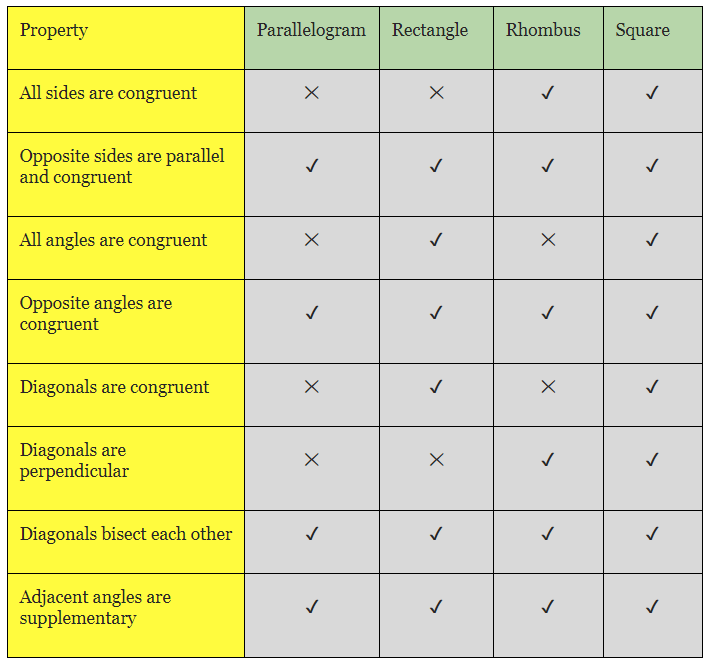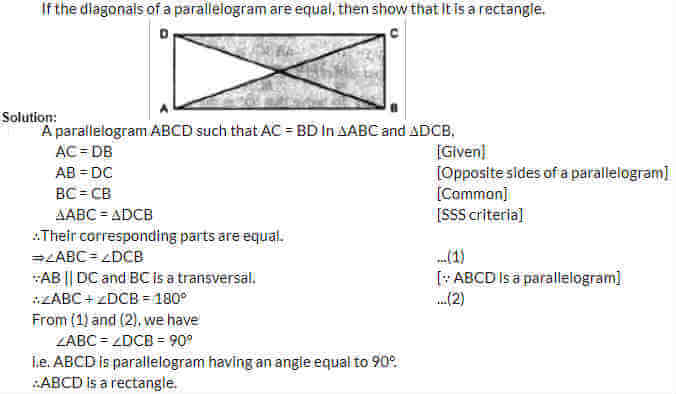# AP Board Class 9 Maths Chapter 8 Quadrilaterals

In AP Board Class 9 Maths Chapter 8, students will mostly learn about convex quadrilaterals. They will study the definition of a quadrilateral, know the different types and understand the properties of quadrilaterals. Some important topics included in this AP Board Class 9 Maths Chapter 8 Quadrilaterals are;

• Properties and Diagonals of Parallelogram
• The Mid-point Theorem of a Triangle

A quadrilateral is a simple closed figure bounded by four lines in a plane or it is any 4-sided polygon. The sum of four angles in a quadrilateral is 360 degree or 4 right angles. It is also known by other names like Quadrangle or Tetragon. There are several types of or quadrilateral shapes namely;

• Square
• Rectangle
• Parallelogram
• Trapezoid
• Rhombus
• Kite

### Properties of A Convex Quadrilateral

Some of the properties of quadrilaterals include;

1. All interior angles are less than 180° or they add to 360°.
2. The two diagonals both lie inside the quadrilateral.
3. It has four sides or edges.
4. It has four vertices or corners.### Mid-point Theorem Of Triangle And Converse

Theorem 1: The midpoints of two sides of a triangle which is joined by a line segment joining is parallel to the third side and also half of it.

Theorem 2: The line passing through the midpoint of one of the sides of a triangle which is also parallel to another side will bisect the third side.

Students can go through some of the chapter questions along with their solutions given below and know more about the concepts.

Question 1: The angles of a quadrilateral are in the ratio 2:4:8:10. Find the angles.

Solution: Let the angles of the quadrilateral be 2n, 4n, 8n and 10n.

∵ Sum of all the angles of a quadrilateral = 360o

∴ 2n + 4n + 8n + 10n = 360o

= 20n = 360o

We get $x=\frac{360}{24}$

= 15o

∴ 2n = 2 × 15o = 30o

4n = 4 × 15o= 60o

8n = 8 × 15o = 120o

10n = 10 × 15o = 150o

Question 2:Stay tuned to BYJU’s to know more about AP Board and access various study materials, including syllabus, exam timetable, and others.